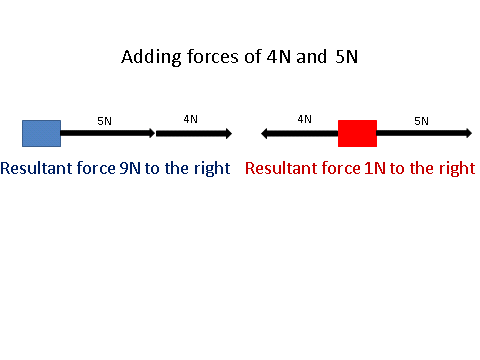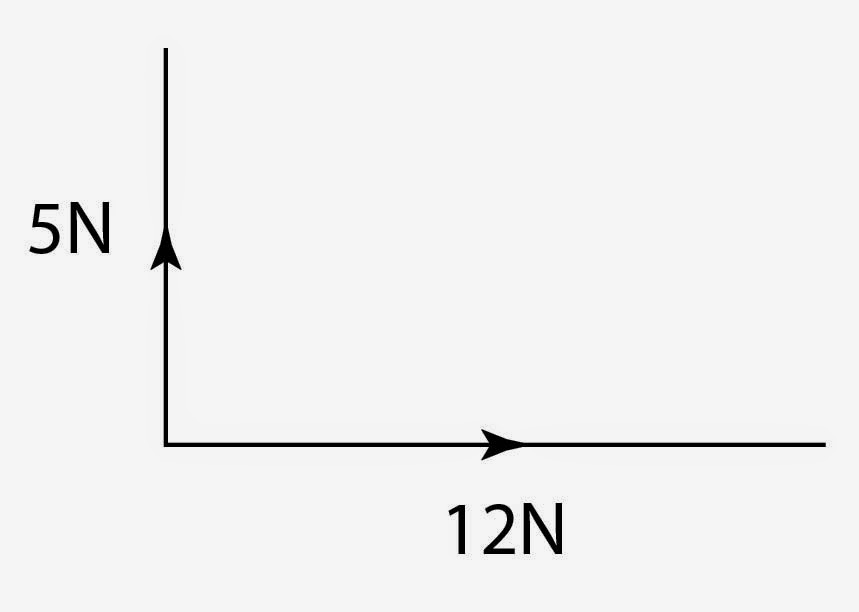# Drawing Forces

## Vector Diagrams

As mentioned previously, all forces are __vector __quantities. Since this is the case, we use __arrows __to show how large the force is and which direction it is acting in.

There are cases in which forces are acting in different directions.Since forces are vectors, you can add them up or subtract them from each other. You can only do this for forces that are acting on the same plane, so vertically or horizontally.

The overall force is called the resultant force. In the case of the blue box, the resultant force is 9N to the right and the red box is 1N to the right, since 5 - 4 = 1.

## Resultant Forces

As mentioned in a previous lesson, the resultant force is the overall force acting on an object. This is where all the forces can be__ replaced by a single force__ that has the exact same effect as all the original forces acting together.

We need to discuss what happens if all of the forces are balanced, which means the resultant force is 0N.

If this is the case, where there is no resultant force, we can say that the object is moving at a constant velocity. To phrase another way, the object is either moving at a constant speed or is stationary (not moving).

Some of the more complicated resultant forces occur when the forces are acting at right angles to each other.

In the example here, the resultant force is a diagonal force pointing top right.To find the value of this force you have to draw these forces to scale!

For example, the 5N might be 5cm and the 12N is 12cm.

We then draw on of the forces, say the 12N and then we draw the 5N going upwards.

You can then measure the distance between the the __start of the 12N line and the end of the 5N line __to get your answer. This means that you MUST __bring a ruler __to the exam!!

The same can be done the other way around. If you are given a diagonal line and are asked to write down the vertical and the horizontal components of the vector then draw the respective lines and measure them. Take heed of any scale factor that is given!

## Lubrication

Lubrication is a way of r__educing the amount of energy wasted__. Normally, wasted energy dissipates to the surroundings via friction __which causes heat. Lubrication helps to reduce friction, hence reduce the amount of wasted energy. This can make a system __more efficient.

﻿

By drawing a diagram, find the resultant force for an object which has a vertical component upwards of 5N and a horizontal component of 8N to the left
9.4N
By drawing a diagram, find the resultant force for an object which has a vertical component upwards of 7N and a horizontal component of 8N to the left and 4N to the right
8N